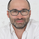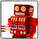4092 views
This is a second attempt at an easy to understand multiple time frame strategy. This one uses ATR for exits. If the position is long, and the price closes below the ATR multiplier, it triggers a close. If the position is short, and the price closes above the ATR/multiplier, it triggers a close. This generates a lot of little trades but is useful because it uses multiple time frames along with cutting losses when the ATR disagrees.
Open-source script

In true TradingView spirit, the author of this script has published it open-source, so traders can understand and verify it. Cheers to the author! You may use it for free, but reuse of this code in a publication is governed by House Rules. You can favorite it to use it on a chart.

Want to use this script on a chart?
```//@version=2
strategy("[RichG] Easy MTF Strategy v1.1", overlay=false)

TF_1_time = input("D", "Timeframe 1")
TF_2_time = input("10D", "Timeframe 2")
TF_3_time = input("15D", "Timeframe 3")
TF_4_time = input("30D", "Timeframe 4")
lengthKC=input(20, title="KC Length")
multKC = input(1.5, title="KC MultFactor")
lengthBB=input(20, title="BB Length")
transaction_size = input(100, "Contract/Share Amount")

src = close, len = 20

out = sma(src, len)
width = 5
upcolor = green
downcolor = red
neutralcolor = blue
linestyle = line

kc() =>
ma = sma(close, lengthKC)
range = tr
rangema = sma(range, lengthKC)
upperKC = ma + rangema * multKC
lowerKC = ma - rangema * multKC
[lowerKC, upperKC]

bb() =>
source = close
basis = sma(source, lengthBB)
dev = multKC * stdev(source, lengthBB)
upperBB = basis + dev
lowerBB = basis - dev
[upperBB, lowerBB]

TF_1 = security(tickerid, TF_1_time, open) < security(tickerid, TF_1_time, close) ? true:false
TF_1_color = TF_1 ? upcolor:downcolor

TF_2 = security(tickerid, TF_2_time, open) < security(tickerid, TF_2_time, close) ? true:false
TF_2_color = TF_2 ? upcolor:downcolor

TF_3 = security(tickerid, TF_3_time, open) < security(tickerid, TF_3_time, close) ? true:false
TF_3_color = TF_3 ? upcolor:downcolor

TF_4 = security(tickerid, TF_4_time, open) < security(tickerid, TF_4_time, close) ? true:false
TF_4_color = TF_4 ? upcolor:downcolor

TF_global = TF_1 and TF_2 and TF_3 and TF_4
TF_global_bear = TF_1 == false and TF_2 == false and TF_3 == false and TF_4 == false
TF_global_color = TF_global ? green : TF_global_bear ? red : white
TF_trigger_width = TF_global ? 6 : width

plot(1, style=linestyle, linewidth=width, color=TF_1_color)
plot(5, style=linestyle, linewidth=width, color=TF_2_color)
plot(10, style=linestyle, linewidth=width, color=TF_3_color)
plot(15, style=linestyle, linewidth=width, color=TF_4_color)
plot(25, style=linestyle, linewidth=4, color=TF_global_color)

exitCondition_Long = TF_global_bear
exitCondition_Short = TF_global

longCondition = TF_global
if (longCondition)
strategy.entry("MTF_Long", strategy.long, qty=transaction_size)

shortCondition = TF_global_bear
if (shortCondition)
strategy.entry("MTF_Short", strategy.short, qty=transaction_size)

[kc_lower,kc_upper] = kc()

strategy.close("MTF_Long", when=close < kc_upper)
strategy.close("MTF_Short", when=close > kc_lower)
```